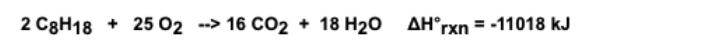# Problem: Using the following equation for the combustion of octane, calculate the amount of moles of carbon dioxide formed from 100.0 g of octane. The molar mass of octane is 114.22 g/mol. The molar mass of carbon dioxide is 44.01 g/mol.a. 6.997 molesb. 8.000 molesc. 10.93 molesd. 18.18 molese. 14.00 moles

###### FREE Expert Solution
95% (281 ratings)
###### FREE Expert Solution

Given:

• mass octane (C8H18) = 100 g
• molar mass octane (C8H18) = 114.22 g/mol
• molar mass carbon dioxide (CO2) = 44.01 g/mol  (extra information, not needed)

95% (281 ratings)###### Problem Details

Using the following equation for the combustion of octane, calculate the amount of moles of carbon dioxide formed from 100.0 g of octane. The molar mass of octane is 114.22 g/mol. The molar mass of carbon dioxide is 44.01 g/mol.

a. 6.997 moles

b. 8.000 moles

c. 10.93 moles

d. 18.18 moles

e. 14.00 molesWhat scientific concept do you need to know in order to solve this problem?

Our tutors have indicated that to solve this problem you will need to apply the Stoichiometry concept. You can view video lessons to learn Stoichiometry. Or if you need more Stoichiometry practice, you can also practice Stoichiometry practice problems.

What is the difficulty of this problem?

Our tutors rated the difficulty ofUsing the following equation for the combustion of octane, c...as low difficulty.

How long does this problem take to solve?

Our expert Chemistry tutor, Sabrina took 3 minutes undefined to solve this problem. You can follow their steps in the video explanation above.

What professor is this problem relevant for?

Based on our data, we think this problem is relevant for Professor Jenkins' class at GGC.